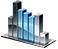# Enumerators

Enumerators are used for creating a list of integer constants. To create an enumerator the following syntax should be used:

` enum listing_name { identifier_1 = value_1, identifier_2 = value_2, ... } `

"value_1", "value_2" are optional. In case the programmer does not specify those values the following rules may be applied:

• Values are assigned sequentially. The first identifier is assigned to 0, the second - 1, etc.
• If the previous identifier is assigned to a certain value the next identifier will be assigned to the "value"+1

Example:

` enum MyEnum { eValue1, eValue2 = 2, eValue3, eValue4 = eValue2 * 100 } int Initialize() { return(0); } int Run() { System.Print("eValue1 = "+ int(eValue1)); // prints eValue1 = 0 System.Print("eValue2 = "+ int(eValue2)); // prints eValue2 = 2 System.Print("eValue3 = "+ int(eValue3)); // prints eValue3 = 3 System.Print("eValue4 = "+ int(eValue4)); // prints eValue4 = 200 return (0); } int DeInitialize() { return(0); } `